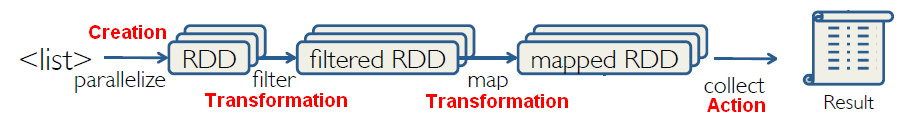# Python - lambda functions

Language - Lambda function in Python.

## Syntax

The expression below yields a function object.

````lambda arguments: expression `
```

The unnamed object behaves like a function object defined with

``````def name(arguments):
return expression```
```

lambda generates a function and returns it, while def generates a function and assigns it to a name.

## Type

``````addSLambda = lambda x: x + 's'
```
``````<type 'function'>
<function <lambda> at 0xb0ef96bc>
nicos
```
```

## Argument

Lambda expressions can be used to generate functions that take in zero or more parameters.

The syntax for lambda allows for multiple ways to define the same function.

For example:

• the parameter is a tuple consisting of two values, and the function adds the two values together. The second lambda relies on the tuple being unpacked automatically.
``````lambda x: x + x
#or
lambda (x0, x1): x0 + x1```
```
• the parameter is two tuples (1, 2) and (3, 4) and the function adds the values element wise (4, 6)
``````lambda x, y: (x + y, x + y)
lambda (x0, x1), (y0, y1): (x0 + y0, x1 + y1)```
```

The result of applying either of these functions to tuples (1, 2) and (3, 4) would be the tuple (4, 6).

Full Example

``````# One-parameter function
a1 = lambda x: x + x
a2 = lambda (x0, x1): x0 + x1
print 'a1( (3,4) ) = {0}'.format( a1( (3,4) ) )
print 'a2( (3,4) ) = {0}'.format( a2( (3,4) ) )

# Two-parameter function
b1 = lambda x, y: (x + y, x + y)
b2 = lambda (x0, x1), (y0, y1): (x0 + y0, x1 + y1)
print 'b1( (1,2), (3,4) ) = {0}'.format( b1( (1,2), (3,4) ) )
print 'b2( (1,2), (3,4) ) = {0}'.format( b2( (1,2), (3,4) ) )```
```
``````a1( (3,4) ) = 7
a2( (3,4) ) = 7

b1( (1,2), (3,4) ) = (4, 6)
b2( (1,2), (3,4) ) = (4, 6)
```
```

## Restriction

### single logical line

Lambda expressions consist of a single expression statement and cannot contain other simple statements. In short, this means that the lambda expression needs to evaluate to a value and exist on a single logical line. If more complex logic is necessary, use def in place of lambda.

### evaluation to a value

Expression statements evaluate to a value (sometimes that value is None). Lambda expressions automatically return the value of their expression statement. In fact, a return statement in a lambda would raise a SyntaxError.

### Statement not allowed

The following Python keywords refer to simple statements that cannot be used in a lambda expression:

• assert,
• pass,
• del,
• print,
• return,
• yield,
• raise,
• break,
• continue,
• import,
• global,
• and exec.

Also, note that assignment statements (=) and augmented assignment statements (e.g. +=) cannot be used either.

## Example

### Sum

Sum of two arguments, a and b.

``````add = lambda a, b: a + b
```

### If

If in Lambda is a ternary expression

````lambda (x,y): x if y is None else y`
```

## Documentation / Reference

Recommended PagesPython - (Function|Procedure|definition)

in Python. 3155PEP 3155 Qualified name for classes and functions. A function in Python has a type. SeePython - Functional Programming

functional programming in python. Functions: , , . Implementation of map, filter and sum with lambda expression Since the methods for map and filter in the FunctionalWrapper class...Spark - (Map|flatMap)

The map implementation in Spark of map reduce. map(func) returns a new distributed data set that's formed by passing each element of the source through a function. flatMap(func) similar to map but...Spark - pyspark

pyspark is the Spark Python API It's also the name of a the pyspark command client We can use lambda functions wherever function objects are required, but they're restricted to a single expression....